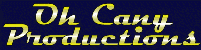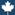Oh Cany Blog
Happy Pi Day!!

Oh Number PI

Oh, number Pi
Oh, number Pi
Your digits are unending,
Oh, number Pi
Oh, number Pi
No pattern are you sending.
You're three point one four one five nine,
And even more if we had time,
Oh, number Pi
Oh, number Pi
For circle lengths unbending.

Oh, number Pi
Oh, number Pi
You are a number very sweet,
Oh, number Pi
Oh, number Pi
Your uses are so very neat.
There's 2 Pi r and Pi r squared,
A half a circle and you're there,
Oh, number Pi
Oh, number Pi
We know that Pi's a tasty treat.

 Posted By: Pete Email: pberg1@ithaca.edu Date: Sunday, March 14 2004 Time: 17:47